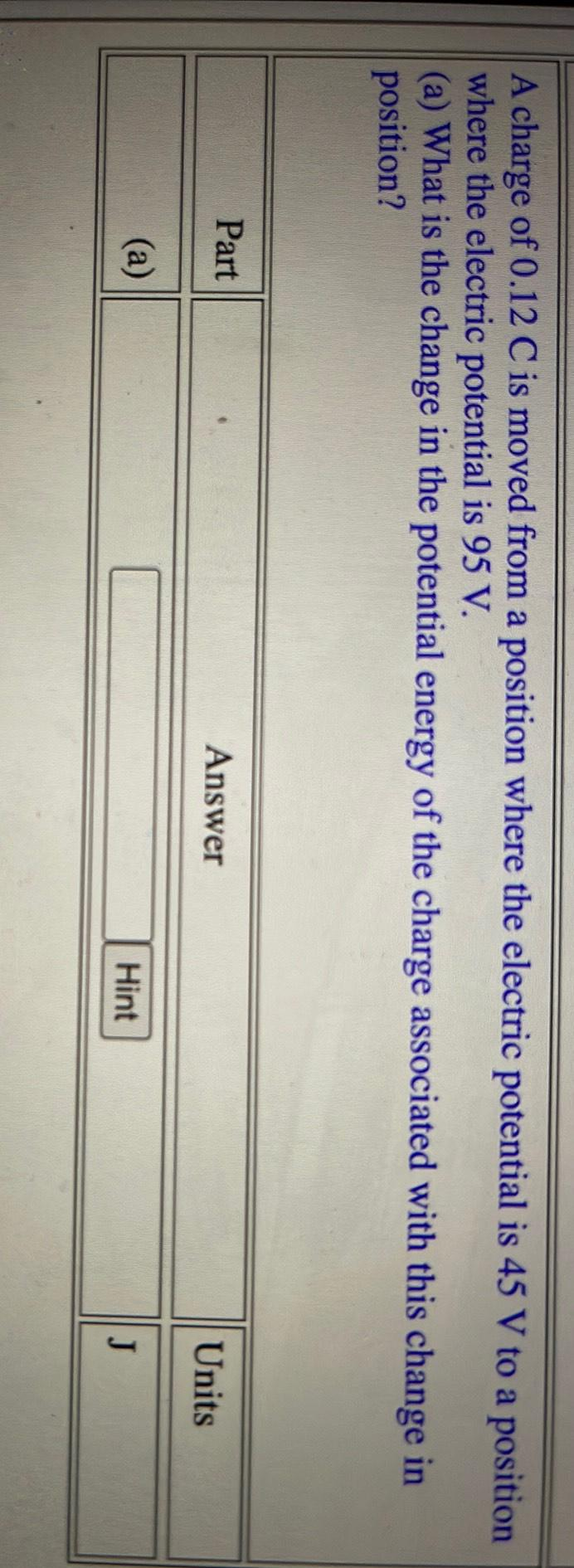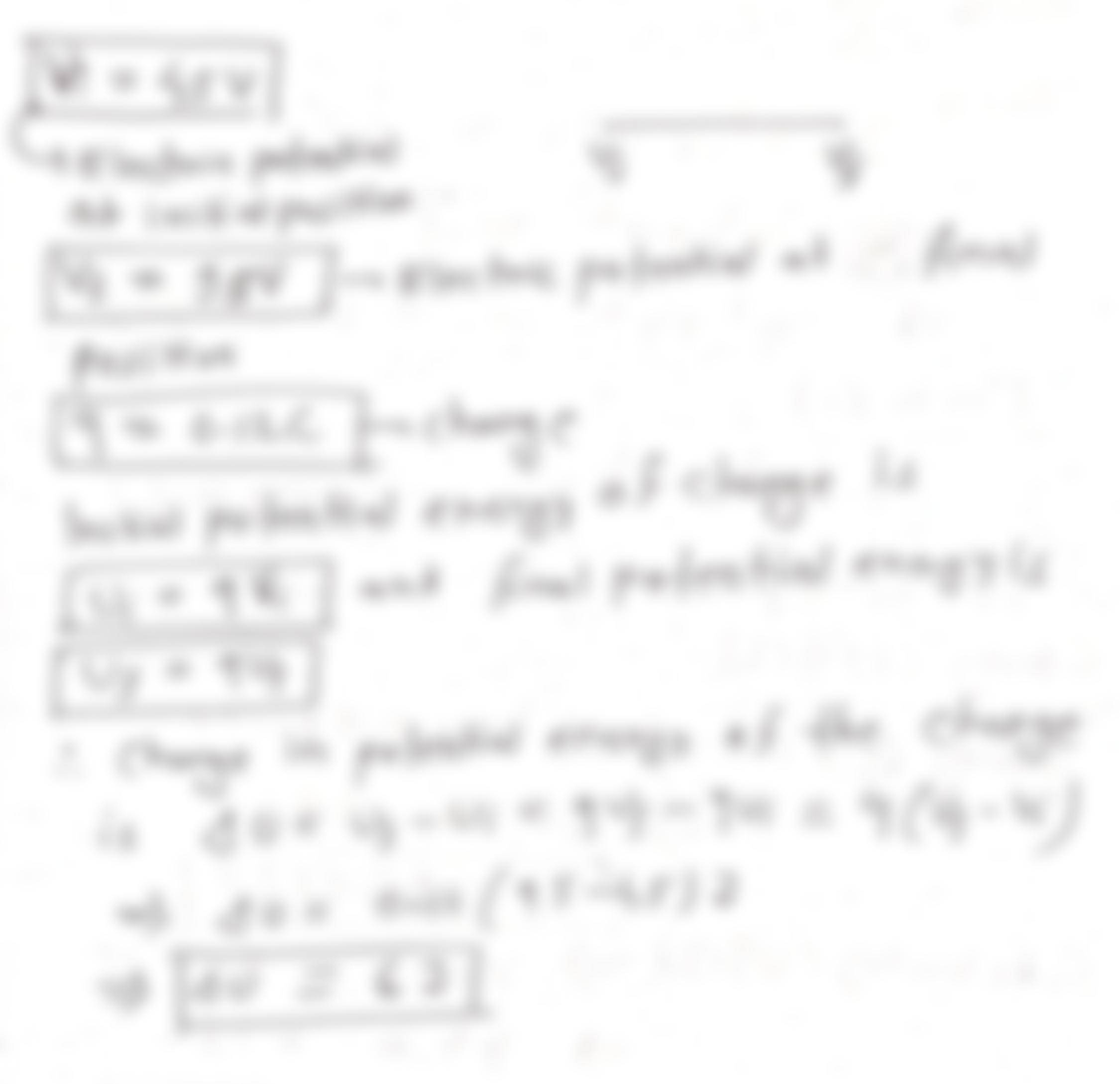Question:

A charge of 0.12 C is moved from a position where the electric potential is 45 V to a position where the electric potential is 9A charge of 0.12 C is moved from a position where the electric potential is 45 V to a position where the electric potential is 95 V. (a) What is the change in the potential energy of the charge associated with this change in position? Part Answer Units (a) Hint J